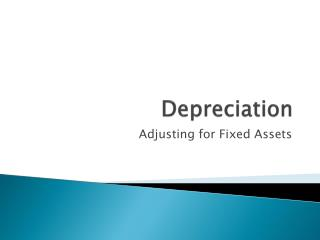DownloadDownload PresentationDepreciation

# Depreciation

Download Presentation## Depreciation

- - - - - - - - - - - - - - - - - - - - - - - - - - - E N D - - - - - - - - - - - - - - - - - - - - - - - - - - -
##### Presentation Transcript

1. Depreciation Adjusting for Fixed Assets

2. Depreciation • Allocation of the cost of a fixed asset over the time it is used to earn revenue • Part of the cost of purchasing a fixed asset is an expense of every period where it is used • Cost has to be spread over the time the assets and has nothing to do with a drop in market value of an asset

3. Calculating Depreciation • Not an exact calculation • Don’t know the exact life of an asset until the asset is not longer in use • Depreciation is based on a “best guess” estimate of useful life

4. Methods of Depreciation • Straight line depreciation where the amount of depreciation is the same from year to year • Capital Cost Allowance method required by Revenue Canada for the amount of depreciation used in calculating income tax.

5. Straight-line Depreciation • Simplest method • Based on a formula Depreciation/yr = original cost – salvage value Estimated years of useful life

6. Sample Calculation We purchase a truck worth \$40 000 with an estimated useful life of 6 years and an estimated salvage value of \$10 000. What is the depreciation for one year? Dep’n = Cost-Salvage Years Dep’n = (40 000 – 10 000)/6 = \$5 000/yr

7. Accumulated Depreciation Account • Used to show the amount of the cost of the fixed asset which has been depreciated in prior years • Is a contra asset account • Balance is used to reduce the “book value” of a fixed asset • Is not cash or have anything to do with cash • AKA “valuation account” • Cost of Asset – Accumulated Depreciation gives the true value or book value of asset

8. Putting this together Use the example of a truck costing\$40 000, estimated to last for 6 years, and with a salvage value of \$10 000. Dep’n = (40 000 – 10 000)/6 = \$5 000 per year The book value of the truck after one year is cost of truck – accumulated depreciation. BV (book value) = 40 000 – 5 000 = 35 000

9. Adjusting Entry Depreciation Expense, Truck \$5 000 Accumulated Depreciation, Truck \$5000 It is customary to abbreviate the account titles using Dep’n for Depreciation and Acc. for accumulated.

10. Other fixed assets • All fixed assets except land are subject to depreciation • Entry for Depreciation is Dep’nExpense, Asset xxxx Acc. Dep’n, Asset xxxx Format remains the same, just insert the type of fixed asset

11. Partial years Two methods: • Half year convention—Record half a year’s depreciation in the year you buy a fixed asset no matter what month you buy the asset and another half year’s depreciation when you sell the asset • Based on months in the year • Monthly statements are based on one month

12. Adding Depreciation to the Worksheet

13. Calculating depreciation • Equipment is \$22 000; useful life is 10 years; and salvage value is \$2 000 • Dep’n = (22 000 – 2 000)/10 = \$2 000 • Automobile is \$21 000; useful life is 5 years and salvage value is \$6 000 • Dep’n= (21 000 – 6 000)/5 = \$3 000

14. Adding Depreciation to the Worksheet